# Topic: Determinants (Test 1)

Topic: Determinants
Q.1
Let A =, then Det. A is
A. none of these
B. 0
C. 1+ab+bc+ca
D. ab +bc+ca
Answer : Option B
Explaination / Solution: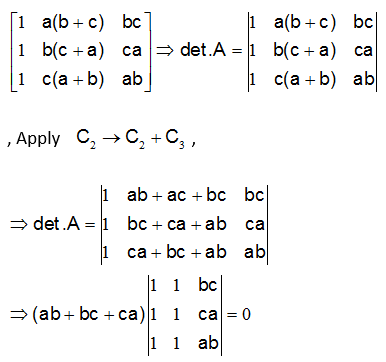( since C1 and C2 are identical)

Workspace
Report
Q.2
The value of the determinantA. 2(x–y)(y–z)(z–x)
B. none of these
C. (x–y)(y–z)(z–x)
D. (x–y)(y–z)(z–x)(x+y+z)
Answer : Option D
Explaination / Solution:Workspace
Report
Q.3A. a+b+c
B. 1+a+b+c
C. abc
D. none of these.
Answer : Option B
Explaination / Solution:Workspace
Report
Q.4A. none of these.
B. x = 2 or 6
C. x = 2
D. x = 4 or 3
Answer : Option D
Explaination / Solution:because , the value of the determinant is zero only when , the two of its rows or column are identical., Which is possible only when Either x = 3 or x = 4 .

Workspace
Report
Q.5
DELETE ITA. 0
B. x3(x+10)
C. None of these
D. (x+10)x2
Answer : Option B
Explaination / Solution: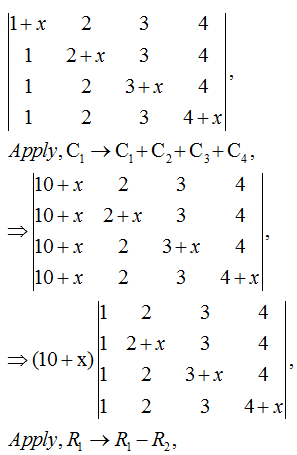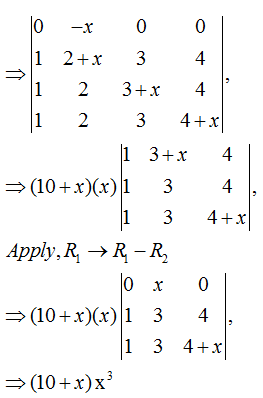Workspace
Report
Q.6A. 0
B. 2
C. -1
D. 1
Answer : Option C
Explaination / Solution:Workspace
Report
Q.7
The roots of the equation det.A. none of these.
B. 1 and 3
C. 2, and 3
D. 1 , 2, and 3
Answer : Option D
Explaination / Solution:

Expanding along C1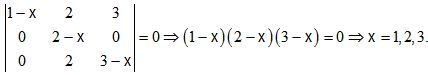Workspace
Report
Q.8
The determinantis equal to
A. 0
B. none of these
C. 1
D. -1
Answer : Option A
Explaination / Solution: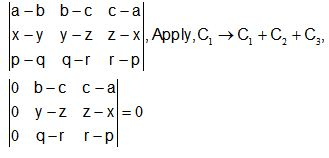(Since C1 =0)

Workspace
Report
Q.9
Find the area of triangle with vertices ( 1 ,1 ) , (2 ,2 ) and ( 3, 3 ).
A. 1
B. 3
C. 0
D. 2
Answer : Option C
Explaination / Solution:

AREA OF TRIANGLE=That is C1 and C2 are identical

so value of determinant =0

hence area of triangle =0

Workspace
Report
Q.10
If A is a square matrix of order 2 , then det (adj A) =
A. A2=O
B. I
C. |A|..
D. 2A2
Answer : Option C
Explaination / Solution:

Let A be a square matrix of order 2 then , because  where n is the order of square matrix.

Workspace
Report